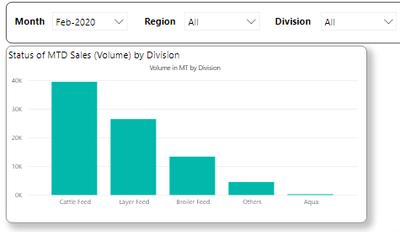cancel
Showing results for
Did you mean:Frequent Visitor

## Total Bar in a clustered column chart

Hi All,

I have created a clustered column chart which has Divisions on the Axis and Volume in Values. The graph is filtered by slicers (I am using Period, Division and Region dimensions for the same).I am trying a add a Total Bar in the same chart as shown below, which should get filtered by the slicer selections.I have tried creating a measure as given below, but it does not work for the selection made using the slicers.

Volume =
var amount = sum(Regional_Forecast_Actual_Table[MT Regional Forecast])
return
if(ISBLANK(amount),
CALCULATE(sum(Regional_Forecast_Actual_Table[MT Regional Forecast]),
ALLEXCEPT(Regional_Forecast_Actual_Table,Regional_Forecast_Actual_Table[Division])), amount
)

I have also tried using the dax formula given below but the same does not work as well
Volume =
var amount = sum(Regional_Forecast_Actual_Table[MT Regional Forecast])
return
if(ISBLANK(amount),
CALCULATE(sum(Regional_Forecast_Actual_Table[MT Regional Forecast]),
ALLEXCEPT(DimensionPeriod, DimensionPeriod[Division])), amount
)
I am somehow not able to achieve the desired result as it needs to also filter using the slicers

Will appreciate if I can find a solution for this.

Regards

Viraj

3 REPLIES 3Super User

Hey @VIrajMP ,

basically, it's not possible to show axis labels that are not present in the column.

To overcomes this, you have to either to think out of the box, and create an "other" member as shown in this article: https://www.sqlbi.com/articles/showing-the-top-5-products-and-others-row/

You can adopt this to show the total instead of the other.

Another approach is to use visuals that provide the feature of showing a Total like the Zebra BI Charts custom visual available from the Appsource custom visual store (be aware that additional licensing fees may be necessary):

Hopefully, this provides some new ideas on how to tackle your challenge.

Regards,

Tom

Did I answer your question? Mark my post as a solution, this will help others!

Proud to be a Super User!
I accept Kudos 😉
Hamburg, GermanyFrequent Visitor

Hi @TomMartens ,

I will surely try and figure out as per your suggestion. Will also check the Zebra Bi Charts to see if it suffices my requirement.

Will get back once I find something close to what I want to achieve.

Regards

VirajCommunity Support

Hi @VIrajMP ,

I suggest you create a new calculated table:

``x = UNION(VALUES(Regional_Forecast_Actual_Table[Division]),ROW("Division","Total"))``

Then create a new measure :

``Measure = VAR A = MAX(x[Division]) RETURN IF(A <>"Total",CALCULATE(SUM(Regional_Forecast_Actual_Table[MT Regional Forecast]),Regional_Forecast_Actual_Table[Division] = A),CALCULATE(SUM(Regional_Forecast_Actual_Table[MT Regional Forecast]),ALLSELECTED(Regional_Forecast_Actual_Table)))``

Use Division column in calculated table for x-axis  and measure for value.

If this post helps, then please consider Accept it as the solution to help the other members find it more quickly.

Best Regards,

Dedmon Dai